GFG App
Open AppBrowser
Continue

We know how to calculate the areas of some standard curves like rectangle, square, trapezium, etc. There are formulas for areas of each of these figures, but in real life, these figures are not always perfect. Sometimes it may happen that we have a figure which looks like a square but is not actually a perfect square, or it may also happen that we come across some completely different figure for which we have to calculate the area. In such cases, integrals help us. Integrals allow us to calculate the areas for arbitrary curves given their equation. Let’s see the process in detail.

### Formula for Area under simple Curves

Integrals can be seen as a sum and definite integrals can be evaluated through the fundamental theorem of calculus. In the figure below, we can see an arbitrary strip whose length is “y” and width is “dx”. “ydx” can be approximated as an area of the shaded rectangle. This area is called the elementary area. As we move from a to b we can keep adding these areas, and we will get the area under of the region ABCD.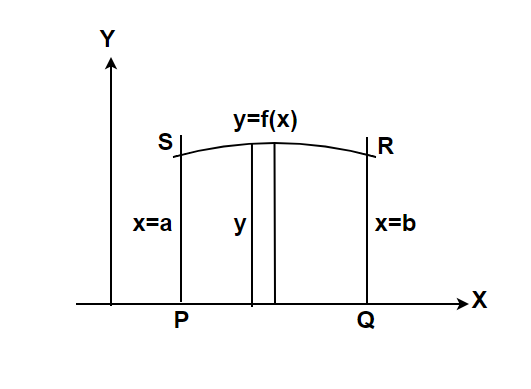So, the area of the region ABCD bounded by the curve f(x) between x = a and x = b is,Similarly, if we have a function g(y) which bounds the area between y = a and y = b. Its area is given by,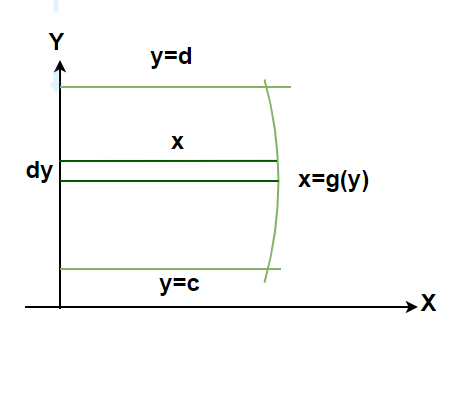Note: If the function is considered to be in the negative interval, then the area comes out to be negative. But we don’t worry about the negative area, it is only numerical value of the area that is taken into consideration.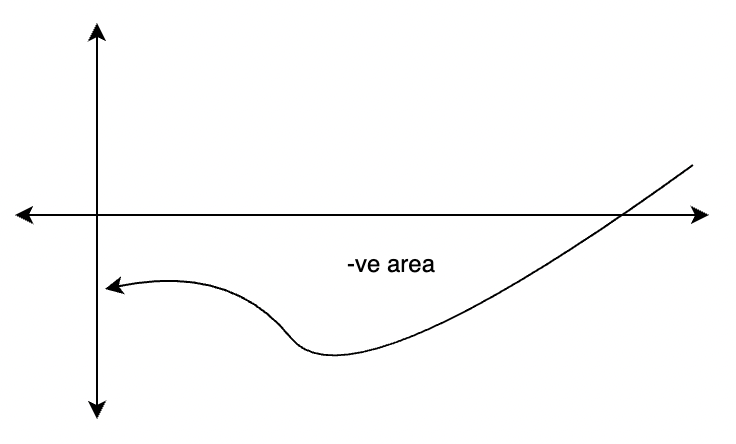Now it might also happen that some part of the area is negative and some is positive as seen in the figure below.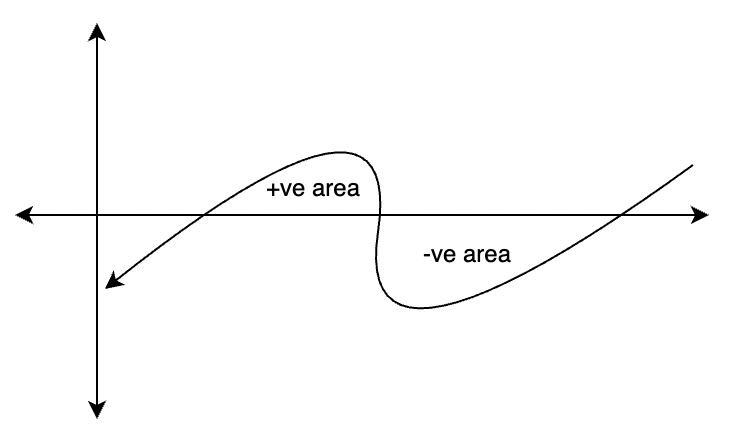The area A1 is negative and A2 is positive. In such cases,

A = |A1| + A2

Let’s see some problems related to this concept

### Sample Problems

Question 1: Find the area enclosed between x = 0 and x = 5 by f(x) = ex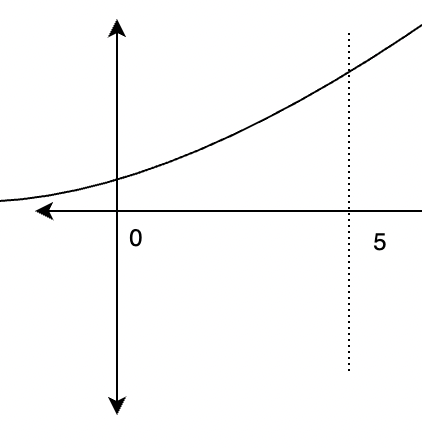Solution:

The area enclosed by the line ===Question 2: Find the area enclosed between the x = 3 and x = 5 by the line y = x.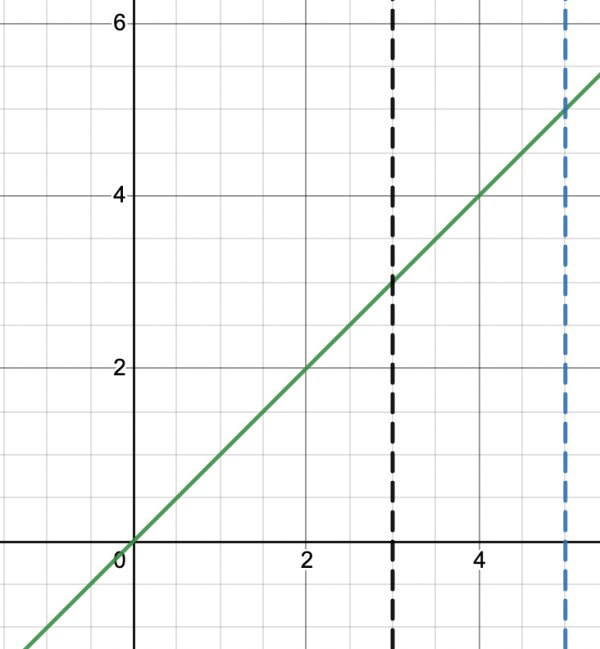Solution:

The area enclosed by the line ===== 8

Question 3: Find the area enclosed by the circle x2 + y2  = b2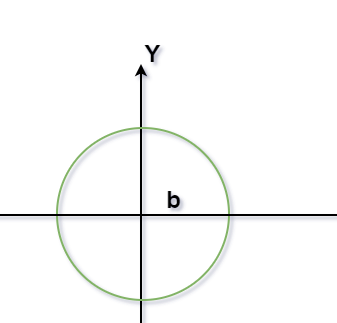Solution:

Figure

The area enclosed by the circle = 4(Area enclosed by the curve between 0 to b)

= 4(Area enclosed in the region POQP)

======Question 4: Find the area enclosed between -2 and 2 for the curve f(x) = x3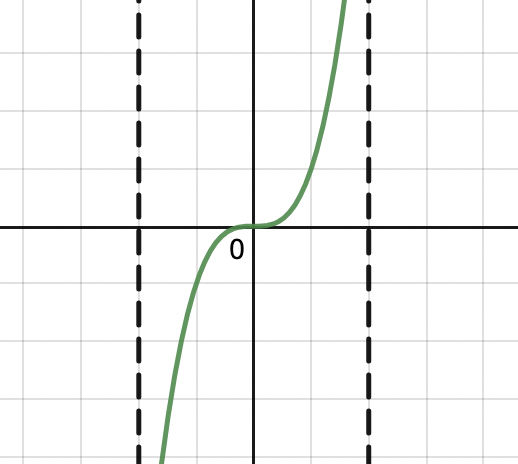Solution:

Given the curve, f(x) = x3. From the graph we can see that half of it’s area is negative, so it will be added with it’s numerical value.

Figure

A ======= 16

Question 5: Find the area of the region that is enclosed between the curve f(x) = x2 and y = 9.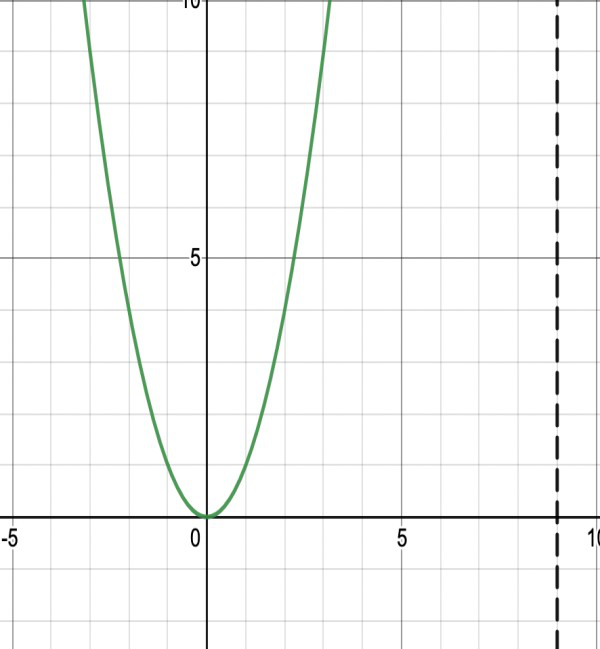Solution:

We can see in the graph that the area of the region enclosed between them will be given by the difference in the area under f(x) and the line y =9.

Figure

The line intersects f(x) at x = 3 and x = -3. So, a = -3 and b = 3.

Let A1 be the area under the f(x)

A1==== 18

Let A2 be the area under the line y = 9.

A2 = 9 (6) = 54

A = A2 – A1

= 54 – 18

= 36

My Personal Notes arrow_drop_up